Filter By

## All Questions

#### To draw a pair of tangents to a circle which are inclined to each other at an Angle of , it is required to draw tangents at end points of those two radii of The circle, the angle between them should be(A)                       (B)                         (C)                       (D)

Solution
According to question:-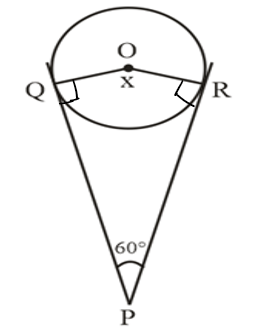Given :
Let
As we know that angle between tangent and radius of a circle is 90

We know that

[ sum of interior angles of quadrilateral is  ]

|

#### To construct a triangle similar to a given  with its sides  of the corresponding sides of  draw a ray BX such that  is an acute angle and X is on the opposite side of A with respect to BC. The minimum number of points to be located at equal distances on ray BX is (A) 5                            (B) 8                            (C) 13                          (D) 3

Solution
To construct a triangle similar to a triangle, with its sides    of the corresponding sides of given triangle, the minimum number of points to be located at an equal distance is equal to the greater of 8 and 5 in  . Here
So, the minimum number of points to be located at equal distance on ray BX is 8.

## Crack CUET with india's "Best Teachers"

• HD Video Lectures
• Unlimited Mock Tests
• Faculty Support#### To construct a triangle similar to a given  with its sides of  the corresponding sides of , first draw a ray BX such that CBX is an acute angle and X lies on the opposite side of A with respect to BC. Then locate points B1, B2, B3, ... on BX at equal distances and next step is to join(A) B10 to C                (B) B3 to C                  (C) B7 to C                  (D) B4 to C

Solution Given:  is an acute angle.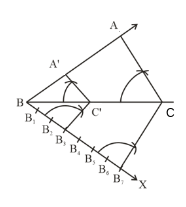Steps of construction
1.   Draw any ray BX making angle with BC on the side opposite to vertex A.
2.   Locate 7 points on BX in equidistant
3.   Now join Bto C

4.   Draw a line through B3  parallel to B7C .

#### To divide a line segment AB in the ratio , draw a ray AX such that  is an acute angle, then draw a ray BY parallel to AX and the points and are located at equal distances on ray AX and BY, respectively. Then the points joined are (A) A5 and B6            (B) A­ and B5               (C) A4 and B5             (D) A5 and B4

Solution

Given:  and  both are acute angles and AX parallel to BY
The required ratio is
Let m = 5 , n = 6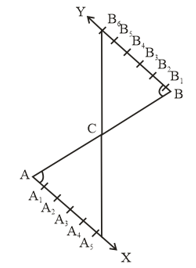Steps of construction
1. Draw any ray AX making an acute angle with AB.
2. Draw a ray .
3. Locate the points  on AX at equal distances
4. Locate the points  on BY at distance equal to the distance between points on AX line.
5. Join .
Let it intersect AB at a point C in figure.
Then
Here   is similar to
Then
by construction

Points joined one A5 and B6.

## Crack NEET with "AI Coach"

• HD Video Lectures
• Unlimited Mock Tests
• Faculty Support#### To divide a line segment AB in the ratio , a ray AX is drawn first such that  is an acute angle and then points  are located at equal distances on the ray AX and the point B is joined to:(A) A12                        (B) A11            (C) A10            (D) A9

Solution

Given:  is an acute angle
The required ratio is
Let m = 4, n = 7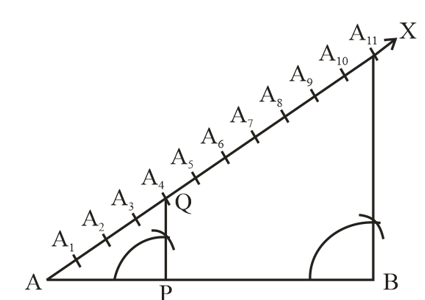Steps of construction
1. Draw any ray AX making an acute angle with AB.
2. Locate 11 points on AX at equal distances   (because m + n = 11)
3. Join
4. Through the point A4 draw a line parallel to   intersecting AB at the point P.
Then

Hence point B is joined to A11.

#### To divide a line segment AB in the ratio , first a ray AX is drawn so that is an acute angle and then at equal distances points are marked on the ray AX such that the minimum number of these points is (A) 8                            (B) 10                          (C) 11                          (D) 12

Solution
Given:  is an acute angle.
The required ratio is
Let m = 5, n = 7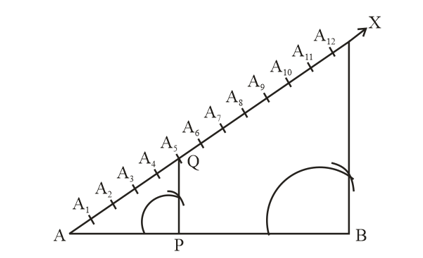Steps of construction
1. Draw any ray AX making an acute angle with AB.
2. Locate 12 points on AX at equal distances.   (Because here )
3. Join
4. Through the point  draw a line parallel to   intersecting AB at the point P.
Then

(By Basic Proportionality theorem)

By construction

Hence the number of points is 12.

## Crack JEE Main with "AI Coach"

• HD Video Lectures
• Unlimited Mock Tests
• Faculty Support#### In Fig. 10.9, AOB = 90º and ABC = 30º, then CAO is equal to: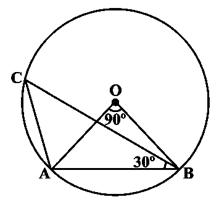(A) 30º(B) 45º(C) 90º(D) 60º

(D)

Solution:In AOB,

OAB +ABO + BOA = 180°      … (i)                           (angle sum property of Triangle)

Angles opposite to equal sides are equal

OAB = ABO

Equation (i) becomes

OAB + OAB + 90° = 180°

2OAB = 180° – 90°

OAB  = 45° …(ii)

In ACB,

ACB + CBA + CAB = 180°   (angle sum property of Triangle)

(Angle subtended at the centre by an arc is twice the angle subtended by it at any part of the circle)

45° + 30° + CAB = 180°

CAB = 180° – 75° = 105°

CAO + OAB = 105°

CAO + 45° = 105°

CAO = 105° – 45° = 60°

Therefore option (D) is correct.

#### In Fig. 10.8, BC is a diameter of the circle and BAO = 60º. Then ADC is equal to: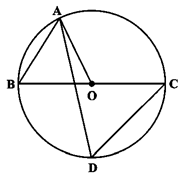Fig. 10.8(A) 30º(B) 45º(C) 60º(D) 120º

(C)

Solution:

In AOB, AO = OB    (Radius)

ABO = BAO         [Angle opposite to equal sides are equal]ABO = 60° [ BAO = 60° Given]

In AOB,

ABO + OAB =AOC (exterior angle is equal to sum of interior opposite angles)

60° + 60° = 120° =AOC

(Angle subtended at the centre by an arc is twice the angle subtended by it at any part of the circle)

Therefore option (C) is correct.

## Crack CUET with india's "Best Teachers"

• HD Video Lectures
• Unlimited Mock Tests
• Faculty Support#### ABCD is a cyclic quadrilateral such that AB is a diameter of the circle circumscribing it and ADC = 140º, then BAC is equal to:(A) 80º(B) 50º(C) 40º(D) 30º

(B) 50°

Solution:

We know that the sum the opposite angles in a cyclic quadrilateral is 180°.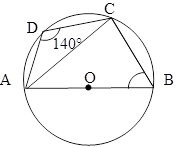140° + ABC = 180°

ABC = 180° – 140°

ABC = 40°

Since, ACB is an angle in semi circle

ACB = 90°

In ABC

BAC + ACB + ABC = 180° (angle sum property of a triangle)

BAC + 90° + 40° = 180°

BAC = 180° – 130° = 50°

Therefore option (B) is correct.

#### In Fig. 10.7, if , then is equal to: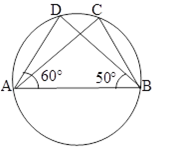Fig. 10.7(A) 60º(B) 50º(C) 70º(D) 80º

(C) 70°

Solution:

In ,

(Angle sum property of triangle)Now,

(Angles in the same segment are equal)

Therefore option (C) is correct.